Courses
Courses for Kids
Free study material
Free LIVE classes
More

# Does It Look The Same Class 5 Notes CBSE Maths Chapter 5 (Free PDF Download)LIVE
Join Vedantu’s FREE Mastercalss

## NCERT Class 5 Maths Chapter 5 Does It Look The Same Revision Notes and Worksheets for Easy Revision and Practice

Students must have studied patterns and learned how to recognize them in the previous classes. The concepts of patterns and their reflections will be lifted to an advanced level in Class 5 Maths Chapter 5. In this chapter, study how the mirror games are played with shapes. To make it easier, download the Does It Look The Same revision notes and start preparing this chapter at your convenience.

Get free worksheets with these revision notes prepared by our subject experts at Vedantu to practice solving the questions of this chapter. Understand the objective from Does It Look The Same summary and focus on developing your problem-solving skills.

Last updated date: 25th Sep 2023
Total views: 143.1k
Views today: 2.43k

## Revision Notes Class 5 Mathematics Chapter 5 - Does it Look the Same?

### Symmetry

• Any Object (Shape, letter or number) that can be divided or folded into two equal halves along a straight line is said to show Symmetry.

• One half of the symmetrical shape is the mirror image of the other.

• Example: Here given both the objects look identical on either side of the line drawn through it.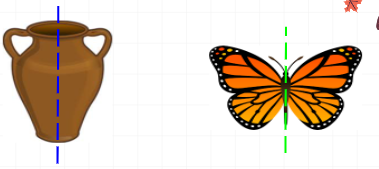Solved Example 1: Name any two alphabets which possess symmetry?

Ans: Alphabet (A), (H) possess symmetry.

Solved Example 2: Can you give any two real objects which possess symmetry?

Ans: Leaf, Lungs in our body possess symmetry.

### Line of Symmetry:

• The line that divides a figure into two equal halves is called the line of symmetry.

• When a figure is folded in half along its symmetry line, the two parts perfectly match.

• For example, we have a tree given here, which we may fold/divide into two equal parts.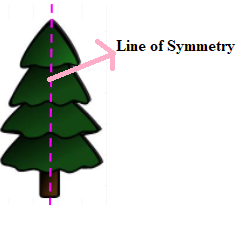• The importance of Line of Symmetry is to make a symmetrical figure, we simply draw the mirror image of the given figure along the line of symmetry.

Solved Example 3: Draw the mirror image of the given figure keeping the line of symmetry in mind.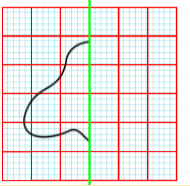Ans: Below given figure shows the full image of the above figure. By using the line of Symmetry concept we have drawn the mirror image of the given half which resulted in the full image.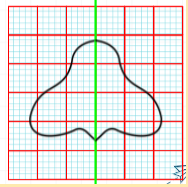### Types of Symmetry:

• There are two types of symmetry. They are (a) Vertical Symmetry and (b) Horizontal Symmetry

• Vertical Symmetry: A vertical line of symmetry is that line which runs down an image thus dividing it into two identical halves.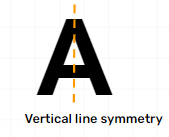• Horizontal Symmetry: A horizontal line of symmetry is that line that runs across the image thus dividing into two identical halves.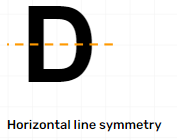• Observing the number of lines of Symmetry: Symmetrical figures can have more than one line of symmetry. The below image shows examples of different number lines of symmetry.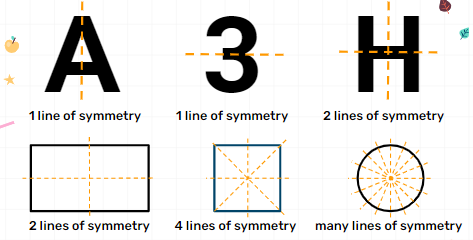### Asymmetric:

• Any Object (Shape, letter or number) that cannot be divided or folded into two equal halves (or) the two sides of any objects are different when compared is called Asymmetric.

• In Asymmetric one half is not a mirror reflection of the other.

• Check out the below image to see the difference between the Symmetry and Asymmetry.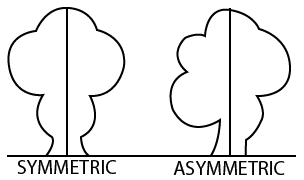Image: Symmetric V/s Asymmetric

### Combination of Symmetry and Asymmetry:

• Objects can be both symmetric and asymmetric, which means even though the shape is Symmetric, the colour scheme/any other difference of the figure can make it Asymmetric.

• For example, here we can observe that with respect to the shape they are symmetric but according to the colour they are asymmetric.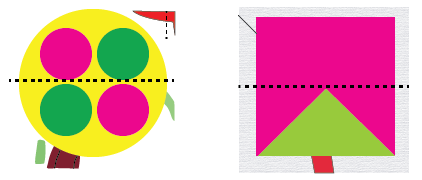Image: Combination of Symmetry and Asymmetry:

### Rotation of Shape:

• When a shape turns clockwise or anticlockwise around a fixed spot the size and the shape itself will remain the same, but its position in space will change.

• Clockwise Direction: It is the same direction as the hands on the clock.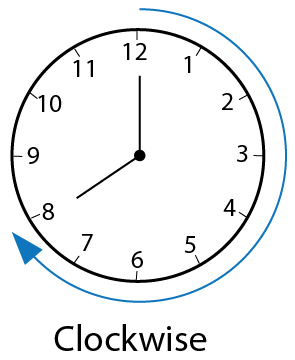Image: Clockwise Motion

• Anti clock Direction: It is the opposite direction in which the hands of a clock move.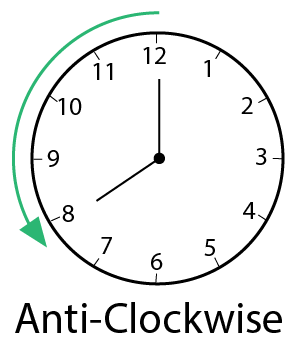Image: Anti- Clockwise direction

• Half Turn: A rotation by 180 degrees (or) 2 right angles (each right angle is 90 degrees) is known as a half turn.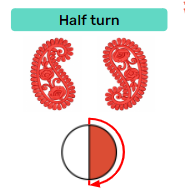• Quarter Turn: A rotation by a right angle i.e, 90 degrees is known as a quarter turn. It is also known as the one-fourth Turn.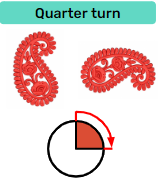• The relation between half a turn and a quarter turn is that 2 quarter Turns  = 1 half turn.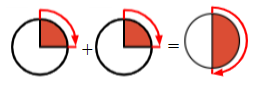• One-Sixth Turn: When an object rotates either clockwise or anticlockwise from point A to point B then we say that the object completed one-sixth rotation.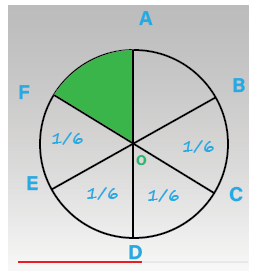Image: Sixth turn

Solved Example 4: Write any 5 examples of 5 digit numbers which look the same on half a turn?

Ans: Give below are the examples 5 digit numbers that look the same on half a turn

10001

10801

16091

16891

19061

Solved Example 5: Can you find out the turns in the patterns shown in the figure.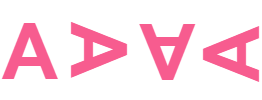Ans: If you clearly observe the diagram it has done one-fourth turn. We all know one-fourth turn is nothing but 90 degrees rotation. Let's apply one-fourth turn to the given alphabet and observe whether it matches the given image or not.

On applying the one-fourth turn we will get the turns in the below image form.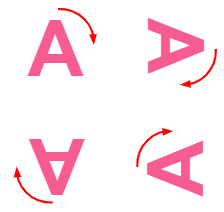Hence the answer is one-fourth turn.

### Patterns:

• Patterns are repeated arrangements of shapes, colours and numbers which are arranged while rotating it. We can create patterns by rotating shapes.

• There are different patterns that we can observe in our daily life, For example, floor pattern, wall patterns, design patterns of sarees, dress shirts etc.,

• Rules to Create Patterns: We can make three different rules to turn it clockwise and can create the pattern.

Rule I: Repeat it with a one-fourth turn

Rule II: Repeat it with a half-turn

Rule III: Repeat it with a three-fourth turn.

### Practise Problems

1. Which of the following numbers looks the same from right to left as well as from left to right?

a) 1234321

b) 126787261

c) 124321

d) 12312

2. How many lines of symmetry do the below figure show?

a) 2

b) 3

c) 6

d) 5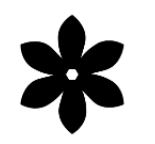## Importance of CBSE Class 5 Maths Chapter 5 Does It Look The Same

• You will be able to recognize different shapes and patterns and their reflections by understanding this chapter. It will teach you how to determine what the shape of an image will become when it is reflected in a mirror. Hence, you will make excellent progress in playing mirror games.

• You will learn how to determine numbers that look the same when they are halved. In this context, numbers with even-digit places will be assigned to check your aptitude.

• Recognize the images of different shapes and objects that will look the same when reflected in a mirror. This chapter focuses on increasing your skills to guess the shapes of different objects and designs.

• Start learning how the images or objects look when they are turned to a certain angle. This chapter teaches how rotating an image will result.

## Benefits of Class 5 Maths Solutions PDF Does It Look The Same

• The revision notes and worksheets are designed following the CBSE guidelines for Class 5. These solutions will also help you to define an effective approach to solving the sums correctly and fast.

• You will also be able to make your pattern based on the questions given in the exercises and worksheets. Follow the simple steps framed by our subject experts to solve these questions and determine the question patterns for better exam preparation.

• The easier explanation of images going through half a turn will guide you to determine the resultant shape. Your imagination power will also increase when you use these PDfs to study and practice the sums of this chapter.

## Download Revision Notes and Worksheets for NCERT Class 5 Maths Chapter 5

Get the free PDFs of Does It Look The Same Class 5 worksheets and revision notes on Vedantu mobile app and official website. Prepare this chapter using these revision notes before your exam, and solve the worksheets at your convenience. Derive better explanations of the fundamental concepts from the notes and clarify doubts on your own.

## Conclusion

The Class 5 CBSE Maths Chapter 5 notes on "Does It Look The Same," available as free PDF downloads, are invaluable educational tools. These notes simplify the complex concept of symmetry, making it accessible and comprehensible for young learners. They offer a structured approach to understanding the symmetry of shapes and objects, promoting clarity in mathematical understanding. Moreover, these notes foster critical thinking and problem-solving skills, which are vital not only in mathematics but also in various aspects of life. The availability of these free PDF notes ensures equitable access to educational resources, facilitating effective learning for all students. In essence, these notes enhance the learning experience, making mathematics engaging and relevant while nurturing a deeper understanding of symmetry in our surroundings.

## FAQs on Does It Look The Same Class 5 Notes CBSE Maths Chapter 5 (Free PDF Download)

1. What is the benefit of using NCERT Solutions for Class 5 Maths Does It Look The Same?

Download and refer to the NCERT Solutions for this chapter, and check how the subject matter experts have framed the solutions. Follow the steps they have used to solve each question and practice accordingly. It will help you to apply your logical reasons for solving such questions.

2.  How can I determine a symmetric object?

Draw a line right through the middle of an image given. Check whether both the halves of this image look similar to each other or not. You might have to rotate an object to find the right middle portion to draw a line.

3.  How can I turn the images to find an answer?

The best way to practice and learn turning images is by drawing them on your copy. Turn the image as per the instructions given in a question and find out the results.

4. Where can I find free PDF downloads of revision notes for Class 5 Maths Chapter 5, "Does It Look The Same"?

You can often find these revision notes on various educational websites and platforms that offer study materials for CBSE Class 5 Mathematics.

5. What is the purpose of revision notes, and how do they differ from regular class notes?

Revision notes are concise summaries of the key concepts and topics covered in a chapter. They are designed to aid in quick revision and review before exams, focusing on essential points.

6. Do these revision notes include solved examples and practice questions for self-assessment?

Revision notes may include solved examples and practice questions to reinforce learning and test comprehension.